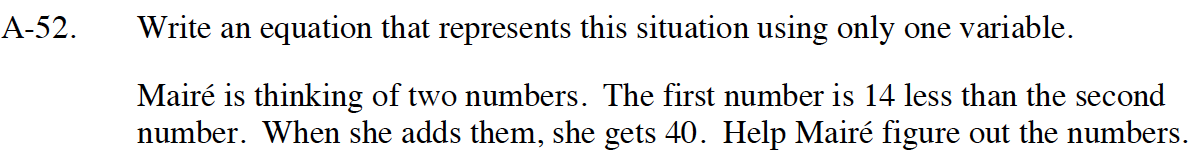Home > CC4 > Chapter A > Lesson A.1.5 > ProblemA-52

A-52.
1. A-52. Write an equation that represents this situation using only one variable.

2. Mairé is thinking of two numbers. The first number is 14 less than the second number. When she adds them, she gets 40. Help Mairé figure out the numbers. Homework Help ✎Let the second number be x. Write an expression for the first number in terms of x.

The equation you need to solve is x + (x − 14) = 40.